Home

# Cubic Functional Equations on Restricted Domains of Lebesgue Measure Zero

## Abstract

Let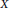$X$ be a real normed space,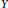$Y$ a Banach space, and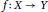$f\,:\,X\,\to \,Y$ . We prove theUlam–Hyers stability theorem for the cubic functional equation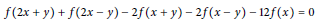$$f\left( 2x\,+\,y \right)\,+\,f\left( 2x\,-\,y \right)\,-\,2f\left( x\,+\,y \right)\,-\,2f\left( x\,-\,y \right)\,-\,12f\left( x \right)\,=\,0$$

in restricted domains. As an application we consider a measure zero stability problem of the inequality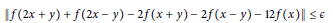$$\left\| f\left( 2x\,+\,y \right)\,+\,f\left( 2x\,-\,y \right)\,-\,2f\left( x\,+\,y \right)\,-\,2f\left( x\,-\,y \right)\,-\,12f\left( x \right) \right\|\,\le \,\varepsilon$$

for all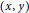$\left( x,\,y \right)$ in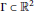$\Gamma \,\subset \,{{\mathbb{R}}^{2}}$ of Lebesgue measure 0.

## References

Hide All
MathJax
MathJax is a JavaScript display engine for mathematics. For more information see http://www.mathjax.org.

# Cubic Functional Equations on Restricted Domains of Lebesgue Measure Zero

## Metrics

### Full text viewsFull text views reflects the number of PDF downloads, PDFs sent to Google Drive, Dropbox and Kindle and HTML full text views.

Total number of HTML views: 0
Total number of PDF views: 0 *Loading metrics...

### Abstract viewsAbstract views reflect the number of visits to the article landing page.

Total abstract views: 0 *Loading metrics...

* Views captured on Cambridge Core between <date>. This data will be updated every 24 hours.

Usage data cannot currently be displayed.